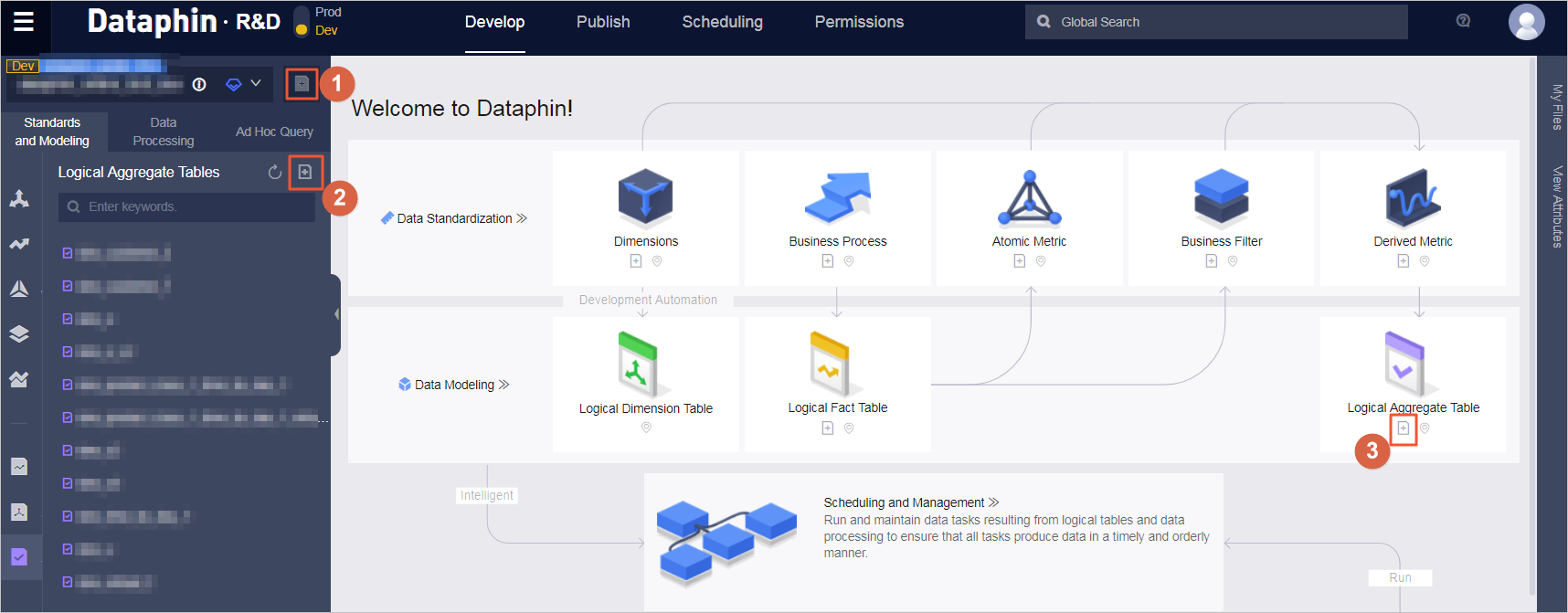A logical aggregate table consists of a specific analysis object (such as a member) and relative statistical metrics. Statistical metrics that constitute a logical aggregate table share the same statistic granularity (such as a member). The aggregate logic provides all statistics data of a theme-based statistic granularity (such as a member). Therefore, Dataphin also supports theme-based data query.

You can collect statistical metrics to form a logical aggregate table with the following two methods: Note: Based on this construction principle, the logical aggregate table only has the Submitted status.
• The system aggregates data based on the same statistic granularity by default.
• According to the same primary key principle, common physical table fields that are created by non-derived metrics are added to the corresponding logical aggregate tables.
1. Choose R&D > Develop > Standards and Modeling > Logical Aggregate Tables to open the corresponding page.
2. You can open the logical aggregate table creation page by clicking one of the three icons in the following figure.
Note After the derived metrics are submitted, a new logical aggregate table is generated by default.3. To create a logical aggregate table, follow these steps: Enter the required information as prompted, click Submit, and then click OK to create a logical aggregate table.